## Complex models and easy interpretability, is that possible?

Started ‎09-06-2022 by
Modified ‎09-06-2022 by
Views 1,028

Using Machine Learning models in our fraud detection systems can be a challenge when it comes to explaining the process or interpreting the results. Having a good triage and alert generation system is key to any good fraud system, but being able to explain correctly and convey to investigators what they need to know to make the right checks is equally important.

Models such as Neural Network or Gradient Boosting give noticeably better results than simpler models, but they can be complicated to explain, and therefore difficult to understand the underlying reasons for why an alert was generated.

To solve this dilemma, there are methods that improve interpretability, and this is what I will briefly explain in this article.

Model-Agnostic methods

One of the key elements to understand these methods is that they are Model-Agnostic, as the name suggests, so let's stop for a moment to see what they are and why they are so beneficial.

The different types of prediction models come with their multiple and complex characteristics and definitions. The Model-Agnostic methods receive that name because they are independent of the type of model, they can be calculated no matter which one you are using since they only use the output of it. Instead of being a middle step in the complex calculations, they are generated after the training of the model using the calculated probability (the final score) and its relationship with the explanatory variables and the objective (input and target).

This means that we can use exactly the same method for all our models, from the most simple to the most complex, and thus gain robustness in our processes and uniformity, as well as simplicity. We don't have to learn multiple methods to make up for the variety of models we have. Therefore, Model-Agnostic methods are our best ally.

Global interpretability vs local interpretability

Once we have seen how beneficial these methods are, there is one more relevant classification for our understanding of the interpretability tools. We can have Global Interpretability methods and Local Interpretability methods.

• Global Interpretability: explains how the model is scoring on average by its characteristics. For example, the variable importance and Partial Dependence Plots, both available in the visual interface for modelling in SAS Viya. Let’s see some examples based on a model that detects if a new transaction is fraudulent or not.
• Variable Importance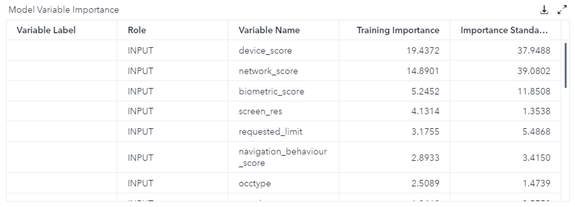Screenshot from Results of a Node in a Machine Learning Pipeline (Build Models tab in SAS Viya)

The Model Variable Importance describes, in order, which of the variables are adding more information to our model from the highest to the lowest. In this example, it would mean that the device_score is the most valuable information we have when figuring out if a new transaction is fraud or not. Following it, the network_score and biometric_score variables are also very important. If we scroll down in the table, we will see the least important variables. You can also check the statistical measures such as the training importance, its standard deviation, and the relative importance.

• Partial Dependence Plots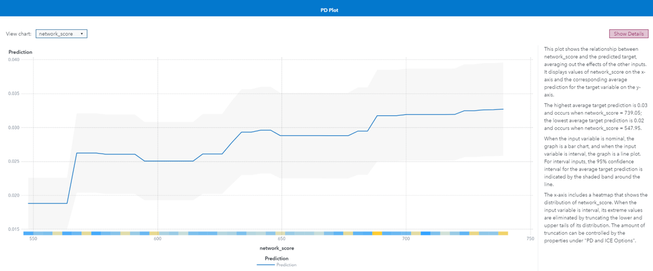Screenshot from Results of a Node in a Machine Learning Pipeline (Build Models tab in SAS Viya)

This is the plot for the input variable ‘Network_Score’, we can have a plot per variable or we could calculate the plots just for the most important ones.

This chart shows the different values of our input versus the final prediction of the model. It's very easy to see in the example that there is a direct relationship between having a higher score (predicted fraud probability) when we have a high ‘Network_Score’. With SAS you can easily create these charts having the advantage of the text explanation, so non-analytical users can interpret it in a more friendly way.

• Local Interpretability: explains how the model is scoring case by case being specific with the characteristics of each case. In these methods we have the Local Interpretable Model-agnostic Explanation (LIME) and Shapley Values. Both create simple models using the input variables and the prediction as a target to have a shortcut result of the weight of the variables in the complex model. The difference between the two methods is that Shapley Values consider the correlation between variables and mathematically and it provides a closer approximation to the weight in the real model, but its implementation is much slower. Both are available in SAS Viya, so let’s see an example with the same model as before.
• LIMEScreenshot from Results of a Node in a Machine Learning Pipeline (Build Models tab in SAS Viya)

In this case we are seeing for that particular transaction (Local Instance), if the variables are making the score increase or decrease.

The variable fraud_on_net has a value of 0 and that is making the score decrease, it contributes negatively as we see with its bar to the left (we can see details with the data tip using a mouse over feature).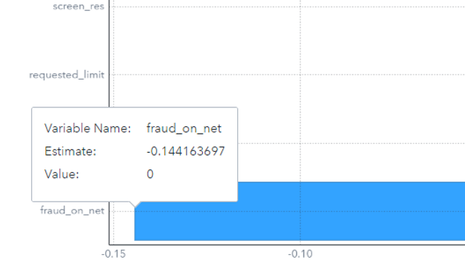Screenshot from Results of a Node in a Machine Learning Pipeline (Build Models tab in SAS Viya)

On the contrary, the device_score has a value of 949, and the estimate is high (and the bar directed to the right), which means that for this observation, the score was highly increased because of a high device_score.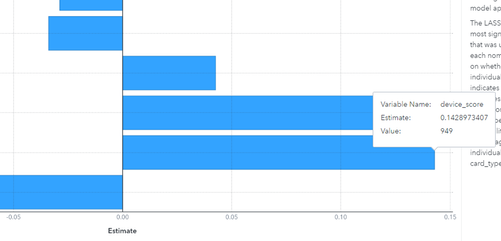Screenshot from Results of a Node in a Machine Learning Pipeline (Build Models tab in SAS Viya)

Following this logic, we can check all the variables and all the transactions, and we would very easily understand the method that our complex model is following.

• Shapley Values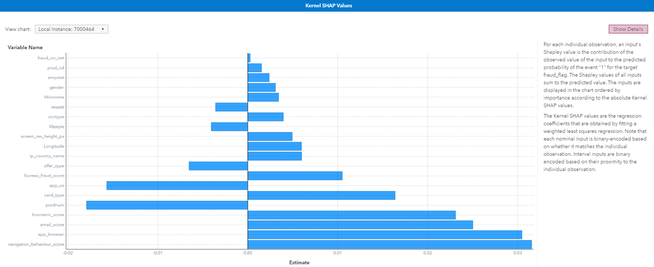Screenshot from Results of a Node in a Machine Learning Pipeline (Build Models tab in SAS Viya)

We can interpret the Shapley Values plot using the same logic as for the LIME, although remember that this calculation is better adjusted and more similar to the model’s algorithm, but more time consuming. We also have some explanation in text to help the correct understanding.

Conclusion

Interpretability methods might be the missing piece of your investigation process. Having great performing models, that are complex to interpret, can be tricky. If you can’t give additional information about alerting events to investigators, apart from the final score, it can lead to a lot of wasted time to find the reason behind a good model.

Although there is much more to learn and investigate, this article provides some options to work around interpretability issues and options to check your scores in an understandable and consistent way. Therefore, you can implement your Machine Learning models without losing the valuable explanations that should go with it.

Version history
Last update:
‎09-06-2022 08:39 AM
Updated by:Contributors
Article Labels
Article Tags